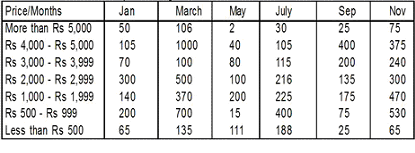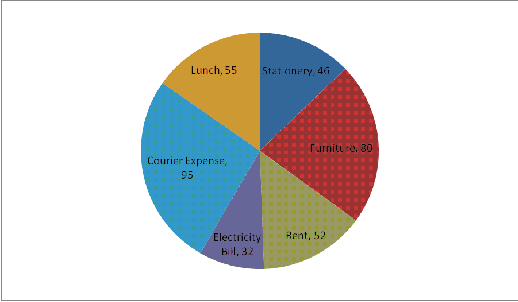# IBPS Quantitative Aptitude model test paper - 7

Home > Aptitude test > Quantitative model test - 7

## IBPS Quantitative Aptitude model test paper - 7

Directions (Q.1-5) What value should come in place of the question mark (?) in the following question?

Q1. √361 + √441 = ?

A. 20
B. 21
C. 40
D. 51

Q2. 1024 ÷ 3 -1014 + 512 – 248 + 1309 = ?

A. 900
B. -90
C. -519
D. 1907

Q3. 2.0105 x 9.99 x 5.14 - ? = - 8923.109

A. 9023
B. 8023
C. 8321.93
D. 9026.345

Q4. 3.14% of 1250 - 1.5% of 2500 = ? – 1.55

A. 1
B. 1.50
C. 3.3
D. 500

Q5. 1/5 of 3/3 of 1/4 of 30000 = ?

A. 1500
B. 150
C. 300
D. 30

Q6. 25 employees can do a piece of work in 20 days. How many employees are required to do a piece of work in 10 days?

A. 40
B. 50
C. 48
D. 45

Q7. (x)1.2 / 54 = 108 / (x) 1.8

A. 18
B. 9
C. 27
D. 81

Q8. The ratio between the present ages of Tanu and Manu is 7:9 respectively. After six years, the ratio of their ages will be 4:5 respectively. What was the age of Tanu before seven years ?

A. 35
B. 40
C. 52
D. 28

Q9. What will come in place of question mark (?) in the following number series?

693 697 706 722 ?

A. 742
B. 750
C. 747
D. 769

Q10. The following questions are accompanied three statement a, b & c. You have to determine which statement(s) is /are necessary sufficient to answer the question?

What is the second number out of third numbers?

a. Sum of the three numbers is 182.
b. The ratio of first and second number is 3:4 and second to third number is 2:3.
c. Middle number is 33.33% greater than the first number and lesser by same percent from third number.

A. Either a and b or a and c.
B. Only a & b.
C. All a, b & c.
D. None of these

ANSWER: A. Either a and b or a and c.

Q11. The following questions are accompanied three statement a, b & c. You have to determine which statement(s) is /are necessary sufficient to answer the question?
How many articles were sold ?

a. Total profit earned was Rs. X
b. Marked Price per article Rs. Y
c. Sold Price per article was Rs. Z

A. Only A
B. Either A or B
C. Only B.
D. None of these.

Directions (Q. 12-16) Study the following table carefully and answer the questions given below:
Number of Cell phones of different ranges bought over the months.Q12. In which month maximum number of cell phones were bought ?

A. July
B. May
C. November
D. March

Q13. In which price range of Rs. 1000 – Rs. 1999 the number of cell phones bought in January is what percent of the number of cell phones bought in May in the same price range?

A.99
B.500
C. 70
D. None of these

Q14. What is the difference between the number of cell phones bought in September and November ?

A. 1020
B. 1620
C. 1775
D. 875

Q15. What is the ratio between the number of cell phones in price range Rs. 4000- Rs. 5000 bought in January and March?

A. 7:100
B. 9:400
C. 21:200
D. None of these

Q16. In which price range maximum cell phones were bought in the given months taken together?

A. Rs. 500-999
B. Rs. 2000-2999
C. Rs. 1000-1999
D. None of these

Directions (Q. 17-18). In the following questions two equations numbered I and II are given. You have to solve both the equations and give answer

1) If a> b
2) If a>= b
3) If a < b
4) If a=5) If a= b or the relationship cannot be established

Q17. I. a2 = -a + 12
II. b2 = 5b – 6.

A. Only 1 follows
B. Only 2 follows
C. Only 5 follows
D. Only 4 follows.

Q18. I. a2 = -11a – 30
II. b2= -7b – 12.

A. Only 1 follows
B. Only 3 follows
C. Only 5 follows
D. Only 4 follows.

Q19. Ajay have ten shirts and twenty five t-shirts in a bag. An apparel is drawn at random. What is the probability of getting a shirt?

A. 1/7
B. 1/10
C. 2/7
D.1

Q20. In how many years a sum of money put out 20% C.I. will be more than doubled ?

A. 5
B. 4
C. 8
D. 7

Directions (Q. 21-25) The circle graph given here shows the spending of an Office on various expenses during a particular year. Study the graph carefully and answer the questions given below

it.
Area of Graph is divided on the basis of Degrees.Q21. What percent of the total spendings is spent on Furniture?

A. 18.50%
B. 13%
C. 24.50%
D. 22.22%

Q22. How much percent more is spent on Lunch than that on Stationery?

A. 16%
B. 25%
C. 33%
D. 50%

Q23. How much percent less is spent on Electricity Bill than on Furniture?

A. 14%
B. 74%
C. 94%
D. None of these

Q24. If the total amount spent on Office during the year was 2 lakh, the amount spent on Furniture and Courier Expense was:

A. 97222
B. 150000
C. 85299
D. 78952

Q25. If the total amount spent on Office during the year be Rs. 1,80,000 , the amount spent on Courier expense exceeds that on Stationery by :

A. 29570
B. 24500
C.44500
D. 74120